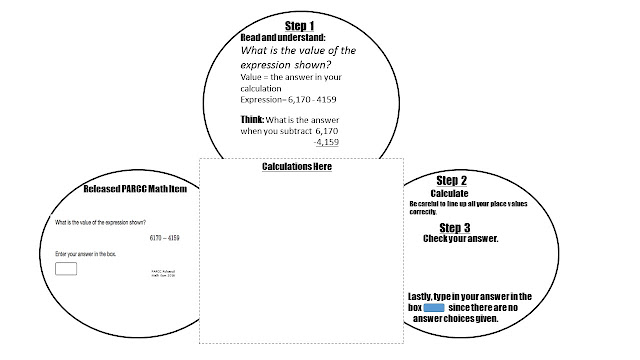Wednesday, December 7, 2016

PARCC Released Math Items 2016: Worksheet to Assist Teachers in Discussing the Items with Students

I believe that I have figured out a way to make it easier for teachers to discuss the PARCC Released Items with their students in an organized way.

For the last 2 days I did a bit of brainstorming and this is my final draft for this newly created fold-able worksheet.Each worksheet will contain a PARCC released math item.
Then, Step-by-Step you can see how you might discuss this item with your students.
Step 1
This area highlights the unique information in the test question that students should understand in order to correctly calculate the mathematics.  On the above page, you can see that the wording of the question might be problematic for some students:
" What is the value of the expression shown?"
The terms value and expression are both explained so that you can discuss this wording with your students in a whole group setting.
Step 2
Calculate.  The area in the center of the fold-able contains the actual calculation. The side circle may give a hint for that calculation.

Step 3
Checking your work or thinking is an important strategy when you are taking a test. Therefore, the "Check Your Answer" space may or may not be used.
Lastly, how to use the computer format is highlighted. After discussing this, the students should complete the answer on the original test item shown on the left circle.

Here is a completed fold-able. As a suggestion, you might want your students to paste the fold-able into a notebook so that just before the actual PARCC test you can review the test taking strategies together. On this example, the student and I summarized what we learned as we looked at this question together.
REMEMBER, I'm only suggesting looking at one problem, and that problem correlates with the math concepts that you are currently studying during math class.
Here are few more examples of fold-ables that I've created so far.

Leave a comment in the comment section below if you would like to have access to these worksheets.
I am planning on making all the finished worksheets for the released item, grouped by Common Core Standard, available at my Teacher Pay Teacher Store, but I'd be glad to share these worksheets with you if you let me know